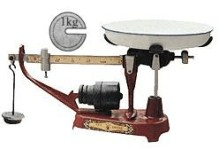$\text{A.}$ 1kg $\text{B.}$ 0.2kg $\text{C.}$ 0.1kg $\text{D.}$ 0.5kg

B